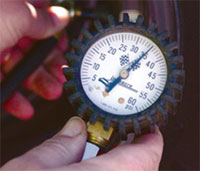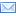#Difference Between Similar Terms and Objects

# Difference Between Gauge pressure and Absolute pressureGauge pressure vs Absolute pressure

Pressure is the force per unit area applied in a direction perpendicular to the surface of an object. Mathematically, it is symbolized with a ‘P’. To put it briefly, it is the amount of force acting on a unit area. The simple formula for pressure is:

P = F / A; where P = pressure
F = force
A = area

The SI unit for pressure is in Pascals (Pa). Other non-SI units are PSI and bar. There are actually many units to express pressure. Every field of science and discipline has different preferences, and it is the same with various regions and organizations.

At times, pressure is expressed as a depth of a particular fluid. The most commonly used are mercury (mm Hg), based on its high density, and water (mm H2O), based on its availability. However, measuring pressure with a column of liquid is not precise. Density of the fluid and especially, gravity can vary in any given region. There are also other kinds of pressure units, such as, ATM and torr.

There are two kinds of references to measure pressure ‘“ the gauge pressure and the absolute pressure. The absolute pressure is measured relative to the absolute zero pressure. Absolute pressure, is pressure that would occur at absolute vacuum, or zero pounds per square inch (PSI). All calculations involving the gas laws require pressure, and temperature, to be in absolute units. Absolute pressure is also referred to as ‘total systems pressure’. To distinguish it from gauge pressure, the term ‘abs’ is usually placed after the unit.

Conversely, gauge pressure is the most commonly used reference of pressure. Machines like air compressors, well pumps, and tire gauges will all use gauge pressure. This pressure reference does not take into account the atmospheric pressure. In other words, gauge pressure uses atmospheric pressure (14.7 PSI), as it’s zero point. Sometimes, it is referred to as ‘overpressure’. A ‘g’ is often placed after the unit of pressure to signify that a particular measurement is a gauge pressure.

It should be noted that atmospheric pressure may vary, depending on many factors, such as locality. Altitude and temperature are essential factors. The standard atmospheric pressure (1 ATM) is about 14.7 PSI.

Summary:

1. Absolute pressure is measured in relation to the vacuum, while gauge pressure is the difference between the absolute pressure and the atmospheric pressure.

2. Absolute pressure uses absolute zero as it’s zero point, while gauge pressure uses atmospheric pressure as it’s zero point.

3. Gauge pressure is commonly used, while absolute pressure is used for scientific experimentations and calculations.

4. To indicate gauge pressure, a ‘g’ is placed after the unit. Absolute pressure, on the other hand, uses the term ‘abs’.

5. Due to varying atmospheric pressure, gauge pressure measurement is not precise, while absolute pressure is always definite.

6. Absolute pressure is sometimes referred to as ‘total systems pressure’, while gauge pressure is sometimes called ‘overpressure’.

Latest posts by Ian (see all)

### Search DifferenceBetween.net :

Custom Search

Help us improve. Rate this post!(13 votes, average: 3.77 out of 5)Loading...Email This Post : If you like this article or our site. Please spread the word. Share it with your friends/family.

1. Relation between absolute and gauge pressure.

What is environment Pressure and how much??

• environment pressure depends on elevation:

at sea level it is equal to 14.7 psia or Zero psig.
once elevation increses atmospheric pressure decreases.
this is one of the reasons why boiling point of water is less than 100 deg. C at a high altitude.